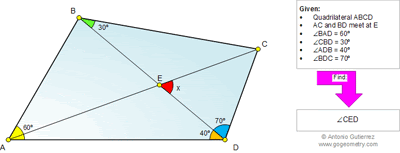Monday, January 13, 2014

Geometry Problem 955: Quadrilateral, Triangle, Diagonals, Triangle, Angles, 30, 40, 60, 70 Degrees

Geometry Problem
Level: Mathematics Education, High School, Honors Geometry, College.

Click the figure below to see the complete problem 955.1.Put A=(0,0), D=(1,0), tan(60)=a, and tan(10)=b.
So, B=((1+ab)/4, (a+3b)/4)
C=((5a+5b-3ab^2-3b^3)/(4(a+2b-ab^2)), (1+b^2)(3-3b^2-2ab)/(4(1-ab)(a+2b-ab^2))
Then, you can find coodinates of E from the above.
(So complicated I skip expression of the results)

Calculate the length of EB, EC, respectively ---> EB=EC.

angle EBC=angle ECB=30 deg.
So x = 60 deg.

2.To Emil
Try to you use elementary geometry (Euclid's Elements). Thanks

1.To use trigonometric functions is violation of rules! I see.
I'll try to find the other solutoin.

3.See following image with comment.
http://postimg.org/image/5arudeby7/full/

4.Draw circumcircle O of triangle ABD, and get P of intersect point of the circle and BC.
Reflect P about AD and get P'.
Triangle APP' is equilateral triangle, since ang.PAD=30deg, that is and.PAP'=60deg.
Triangle P'PC is isoceles, so P'A=P'P=P'C

Ang.ACP=ang.AP'P/2=30deg.

x=ang.DBC+ang.ACB=30deg+30deg=60deg.

5.See attached sketch
http://s3.postimg.org/3ntrf88ur/prob_955.png

Refer to line 4 of your solution " Ang.APB=ang.ADB=40deg, so ang.P'PC=80deg=ang.P'CP "
I don't see the reason for ang. P'CP = 80 deg . Please explain.

6.Construction:
A) Construct point G such that EG is perpendicular to BC and CG is parallel to BD. Label the intersection of EG and BC as F.

B) Construct Altitude triangle ECD, label the intersection with ED as H.

Proof:
1) Angle BEF=60° so Angle HEF=120°=Angle CED

2) Since BD parallel CG, Angle BCG=30° and Angle CGF=60°

3) Angle BEF = Angle CGF; Angle EBF = Angle GCF therefore Triangle BEF is similar to Triangle CGF by AA similarity.

4) From 3 we have:
BF similar to CF
Angle BFE = Angle CFE
EF = EF
Therefore Triangle BFE is similar to Triangle CFE by SAS similarity.
Therefore Angle BEF = Angle CEF = 60°
Therefore Angle CED = 120-60 = 60°
Q.E.D.

7.Construction:

1) Construct EG perpendicular to BC such that CG is parallel to BD, label intersection EG and DC as F.

2) Construct altitude of triangle ECD label the intersection with ED as H.

Proof:

1) Angle BEF=60, so angle HEF=120
2) Since BD parallel CG, angle BCG=30 and angle CGF=60.
3) Angle BEF = angle CGF, and angle EBF = angle GCF, therefore triangle BEF is similar to triangle CGF by Angle Angle similarity.
4) From 3 we have side BF is similar to side CF, angle BFE = angle CFE, EF = EF, therefore triangle BFE is similar to triangle CFE by Side Angle Side similarity.
5) From 4 we have angle BEF = angle CEF = 60, therefore angle CED = 120-60=60. Q.E.D.

1.To Anonymous:
I note that
1. Your proof don’t use given data angle BAD= 60, angle BDA= 40 and angle BDC=70
2. Your steps 4 and 5 state that “4) From 3 we have side BF is similar to side CF, angle BFE = angle CFE, EF = EF, therefore triangle BFE is similar to triangle CFE by Side Angle Side similarity.”
Step 5: “.From 4 we have angle BEF = angle CEF = 60, therefore angle CED = 120-60=60. Q.E.D.”
I am not sure that x can be calculated without using given data. Please explain .

Peter Tran

2.Peter you should try drawing out the constructions. In this case I use angle DBC=30 to get angle BEF=60. With the construction of CG parallel to DB everything else follows. You don't always have to use all of the information provided.

8.Problem 955 revisited.
Please draw a figure
Let B' be the reflection of B in AC.
Let F be the intersection of BB’ with AC.
Angle AB’C = Angle ABC = 120 deg = Angle ADC.
So A,B’,D,C are concyclic
Triangle BB'C is equilateral (since ΔBFC congruent to ΔB'FC)
BE bisects Angle B'BC (since Angle EBC is 30 deg).
Hence x = Angle BEF (complement of Angle EBF) = 60 deg.

1.http://s25.postimg.org/u8npj7cn3/pro_955.png
See attached for the sketch
Referring to line 7 of Pravin’s solution : “Triangle BB'C is equilateral (since ΔBFC congruent to ΔB'FC)”
Note that ΔBFC congruent to ΔB'FC is not enough to conclude that Triangle BB'C is equilateral. Please give more details

2.APOSTOLIS MANOLOUDIS 4 HIGH SHCOOL OF KORRYDALLOS GREECE
Take point K on BD such that AB=AK, with <BAK=20,then AB=AK=KD.Frame the equilateral triangle AKL.
Is AB=AK=AL=KL=KD,therefore A is circumcenter to triangle BKL or <LBK=LBD=1/2<LAK=1/2.60=30=
<DBC. Is K circumcenter to triangle ALD. Therefore <ADL=1/2.<AKL=1/2.60=30.Is <BDL=40+30=70=<BDC.
Therefore triangles BLD and BDC are equal or LD=DC, BC=BL and <LBC=60 then triangle BLC is
E4quilateral or <DCL=20=<DLC=<LAD or ALDC is concyclic then <CAD=<CLD=20.Therefore <CED=x=

9.Very good solution. See below for the sketch of Apotolis's solution

https://goo.gl/photos/2QgHKHkDywG9S91DA

Peter Tran

10.Thank you very much for your nice words about your shape Peter